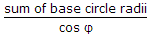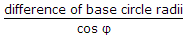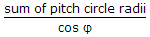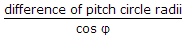# Mechanical Engineering - Theory of machines

### Exercise :: Theory of machines - Section 1

21.

When the two elements of a pair have a surface contact when relative motion takes place and the surface of one element slides over the surface of the other, the pair formed is known as a

 A. lower pair B. higher pair C. self-closed pair D. force-closed pair

Explanation:

No answer description available for this question. Let us discuss.

22.

The centre distance between two meshing involute gears is equal to

 A.B.C.D.Explanation:

No answer description available for this question. Let us discuss.

23.

The two elements of a pair are said to form a __________ when they permit relative motion between them.

 A. open pair B. kinematic pair

Explanation:

No answer description available for this question. Let us discuss.

24.

When the axes of the shafts, over which the gears are mounted, move relative to a fixed axis, then the train is known as reverted gear train.

 A. Right B. Wrong

Explanation:

No answer description available for this question. Let us discuss.

25.

In under damped vibrating system, the amplitude of vibration

 A. decreases linearly with time B. increases linearly with time C. decreases exponentially with time D. increases exponentially with time